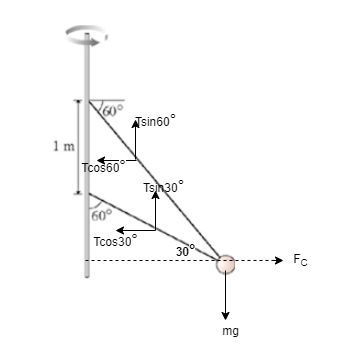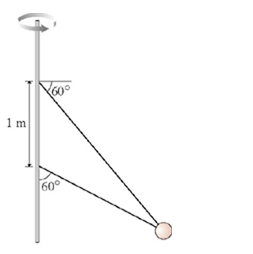# Problem: Two wires are tied to the 3.0 kg sphere shown in the figure. (Figure 1) The sphere revolves in a horizontal circle at constant speed.a) For what speed is the tension the same in both wires?Express your answer to two significant figures and include the appropriate units.b) What is the tension?Express your answer to two significant figures and include the appropriate units.

###### FREE Expert Solution

Net centripetal force:

$\overline{){{\mathbf{F}}}_{{\mathbf{C}}}{\mathbf{=}}\frac{\mathbf{m}{\mathbf{v}}^{\mathbf{2}}}{\mathbf{r}}}$

Consider the free body diagram:a)

Equating the horizontal forces:

T cos (30°) + T cos (60°) = mv2/r

Equating the vertical forces:

T sin (30°) + T sin (60°) = mg

81% (170 ratings)###### Problem Details

Two wires are tied to the 3.0 kg sphere shown in the figure. (Figure 1) The sphere revolves in a horizontal circle at constant speed.a) For what speed is the tension the same in both wires?

Express your answer to two significant figures and include the appropriate units.

b) What is the tension?

Express your answer to two significant figures and include the appropriate units.